### Home > A2C > Chapter 5 > Lesson 5.1.2 > Problem5-28

5-28.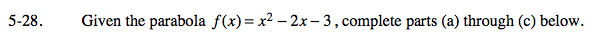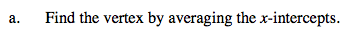Find the x-intercepts by factoring and using the Zero Product Property. Average the two numbers you get. That x-value represents the line of symmetry and the x-coordinate of the vertex.

Substitute the x-value you got into the equation to find the y-coordinate of the vertex.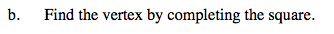What do you need to add to x2 − 2x to make it a perfect square? Remember to add it to both sides of the equation and subtract it from the equation so you keep the equation balanced.

When you rewrite the perfect square in factored form, your equation will be in vertex form: y = a(xh)2 + k, where the vertex is (h, k).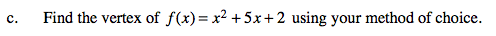(−2.5, −4.25)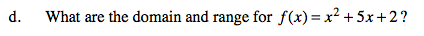Domain: All real numbers
Range: y ≥ −4.25